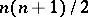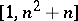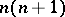# Group of motions

(diff) ← Older revision | Latest revision (diff) | Newer revision → (diff)

A continuous group of transformations of a space, the elements of which are the motions (cf. Motion) of this space, while the group operation is the execution of two motions in a prescribed sequence. In a broad sense, any group of continuous transformations (cf. also Transformation group) of a space may be realized as the group of motions of this space. This is done by introducing, for the given group, a concept of equality of figures, which leads to a new geometry for which this group serves as the group of motions (cf. Erlangen program).

A group of motions is said to be transitive if for two arbitrary points in the space it is possible to select in the group a motion which maps one of the points to the other; it is said to be intransitive if there exists a pair of points for which a selection of such a motion is not possible.

A space into which it is possible to introduce a given group of motions is said to be a space admitting this group of motions. If the group of motions of a space is of the highest order (dimension), it is said to be a complete group of motions. For instance, the complete transitive group of motions of the-dimensional Euclidean space is a group of order, i.e. the group depends onparameters. In the particular case of the Euclidean plane, the parameters of the complete group of motions may be the coordinates of the point into which the coordinate origin is transformed, and the angle of rotation — i.e. this group of motions has three parameters. The group of motionsof the Euclidean plane is a non-commutative solvable group. Its subgroup of rotationsis commutative and intransitive, while the subgroupof parallel transfers is commutative, transitive and is a normal subgroup of the group of motions. The intersection of these two subgroups is the unit of the group of motions. The quotient groupis isomorphic to, and the commutator subgroup ofis contained in. Possible parameters of the group of motions of three-dimensional Euclidean space are the coordinates of the pointinto which the origin is mapped and the Euler angles, i.e. this group of motions is hexaparametric.

Studies on groups of motions in generalized differential-geometric spaces are conducted in several directions at the same time, the most important of which are listed below.

1) The group-theoretic direction : To construct a connection or an invariant metric in the space on which the group of transformations is acting.

2) The study of groups of motions in given spaces, i.e. groups admitted by a space with a given metric or a given connection , .

3) A direction on the interface of the other two, viz. the study of lacunas and lacunary spaces (cf. Lacunary space), i.e. the construction of spaces admitting a complete group of motions of a given order or a demonstration of the non-existence of spaces of a given type which would admit some given group of motions.

For instance, an-dimensional affine space admits a group of motions of maximal order. The order of the groups of motions of other spaces of affine connection belong to the segment of the natural number series, but not every number of this segment can be an order of the complete group of motions.

Intervals of greatest length constituted by numbers that are not orders of complete groups of motions are known as lacunas, and their complements in the series of natural numbers are said to be condensation segments. A space is said to be a space of-th lacunarity if the order of its complete group of motions forms part of the condensation segment with sequence number. A principal problem is the distribution of lacunas and condensation segments of possible orders of complete groups of motions defined for the last groups of motions themselves and for their corresponding lacunary spaces. This problem is closely connected with the study of the degrees of freedom of solid bodies.

Riemannian spaces of constant curvature are the only spaces admitting a complete group of motions of order. In all other cases the group of motions of a space has a smaller number of parameters. A manifold with an affine connection admits a complete group of motions (of order) if and only if the connection is symmetric, while the curvature is equal to zero; in such a case the group of motions is a general linear group.

How to Cite This Entry:
Group of motions. Encyclopedia of Mathematics. URL: http://encyclopediaofmath.org/index.php?title=Group_of_motions&oldid=16960
This article was adapted from an original article by I.P. Egorov (originator), which appeared in Encyclopedia of Mathematics - ISBN 1402006098. See original article